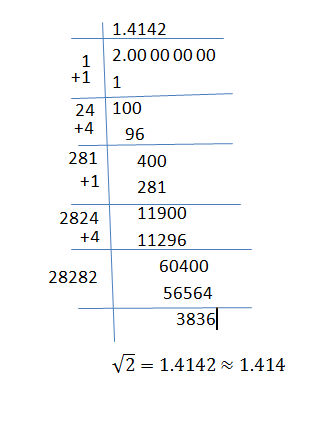Jet Set Go! All about Aeroplanes Jet Set Go! All about Aeroplanes

# Value of Root 2

The square root or root of a number “n” is a number that returns the value equal to “n” when multiplied by itself. For example, the value of the square root of 4 is ±2. Because 2 x 2 = 4. Finding the square root of a number is the inverse process of squaring the number. When we multiply the number to itself, we get the square of a number. Finding the square root of the perfect square number is easier than the non-perfect square numbers.

Examples for perfect square numbers:

4 = square of a number 2 = 2 x 2

9 = square of a number 3 = 3 x 3

16 = square of a number 4 = 4 x 4

25 = square of a number 5 = 5 x 5

It is a bit complicated to find the square root value for the non-perfect square numbers say 10. The symbol used to denote the square root is ‘√’. The square root symbol is also known as a radical symbol or radix. The number underneath the radical symbol is called as radicand. If the given number is not a square number, then the value should take either radical form or decimal form. Finding the value of root 2 is quite difficult since the number 2 is not a square number and there is no such easy method to find the value. The only possible method to find the square root values of the non-perfect square is a long division method.

## Division Method to Find the Value of Root 2

By using the long division method, it is possible to find the value of square roots of any number. The steps for finding the value of root 2 is given below:

Step 1: The number 2 can be written as 2.000000, i.e., 2 = 2.000000

Step 2: Take the perfect square which is below 2. So the perfect square below 2 is 1.

Step 3: Write the number 1 in both divisor and quotient place and subtract 1 from 2, you will get the remainder 1.

Step 4: Next write down two zeros and write down after 1 and take the decimal point after 1 in the quotient.

Step 5: Now add the divisor part with the same number that we have taken already, .i.e. 1, we will get 2 and write down the number in the divisor place opposite to 100. So, after number 2, we have to take the number and the same number has to be taken in the quotient place also. So, when you take the number 4 after 2 and the same number has to be written in the quotient. Because when you multiply 24 with 4, you get 96 which is closer to the number 100. Finally, subtract 96 from 100 and write down the remainder.

Step 6: Now again write down two zero’s and repeat step 5.

Step 7: Finally, you get the quotient value as 1.41421 which is approximately equal to 1.414.So, the value of root 2 is equal to 1.414.

√2 = 1.414

With the help of the long division method, you will find the values of non-perfect square values like root 3, root 5 etc.

Also Check: Value of root 3

Register with BYJU’S – The Learning App to know the root values of other numbers and also watch interactive videos to clarify the doubts.

 Related Links Square Root Table Square Root Calculator Square Root Of 5 Square Root Formula

Test your knowledge on Value of root 2